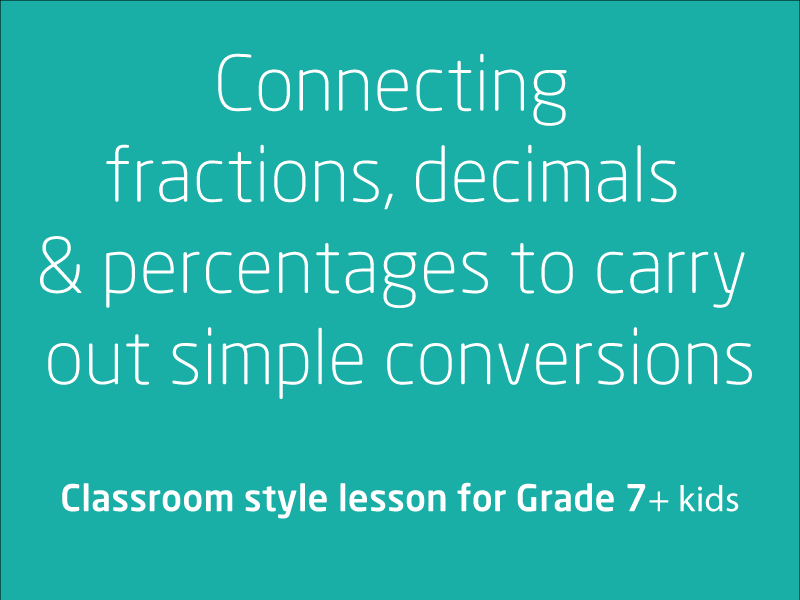# Free video lessons for Foundation to Grade 8 students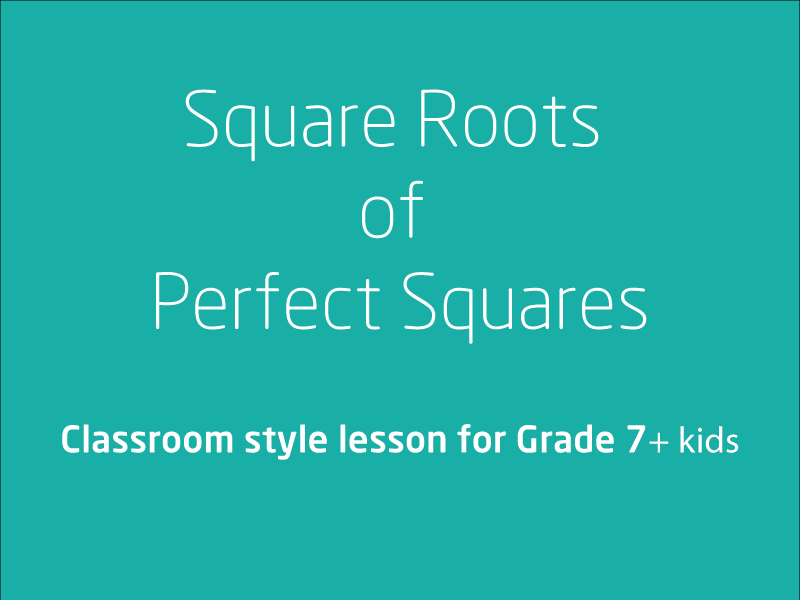### Investigate and use square roots of perfect square numbers

This video lesson is all about learning square roots of perfect squares.Your coach will explain topic in detail with a lot of examples. Practice is the key for you to master this s...

1 videos
Basic difficulty
0 0 0### Index notation & represent whole numbers as products of powers of prime numbers

From this video lesson, you will learn about exponents, prime factorization, prime and composite numbers.ElaborationsElaborationsdefining and comparing prime and composite numbers ...

2 videos
Intermediate difficulty
0 0 0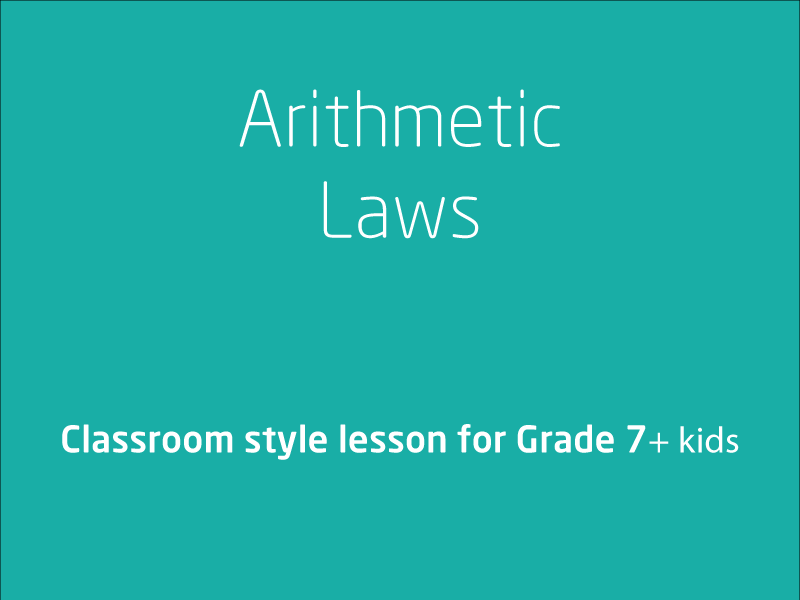### Arithmetic laws

Apply the associative, commutative and distributive laws to aid mental and written computationElaborationsunderstanding that arithmetic laws are powerful w...

1 videos
Intermediate difficulty
0 0 0### Compare, order, add and subtract integers

In this video lesson, your coach will first explain what integers are, that is what qualify as Integers and what isn't an Integer. There are several examples on comparing, orde...

2 videos
Intermediate difficulty
0 0 0### Equivalence fractions

In this video lesson, your coach will teach you how to compare fractions using equivalence. Locate and represent positive and negative fractions and mixed numbers on a number ...

1 videos
Intermediate difficulty
0 0 0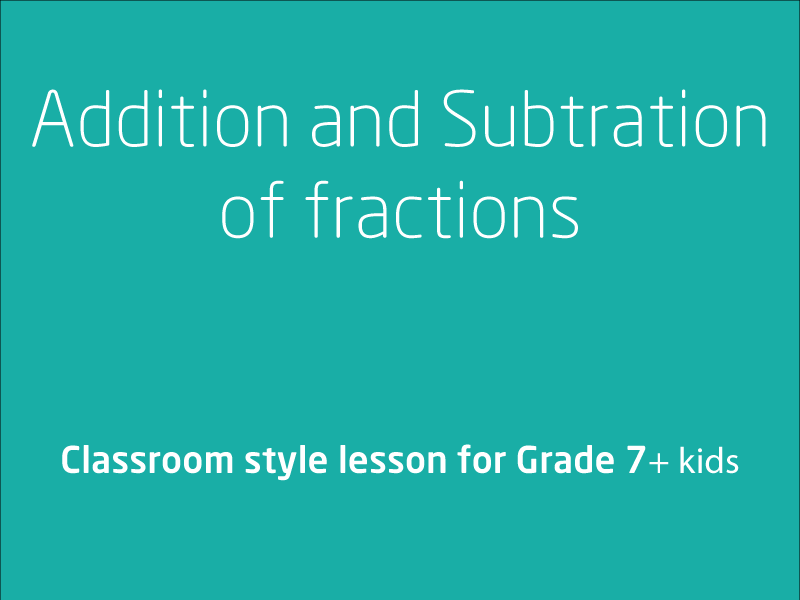### Addition and Subtraction of fractions

In this video lesson we will be looking at solving problems involving addition and subtraction of fractions, including those with unrelated denominators. We will also be explo...

2 videos
Intermediate difficulty
0 0 0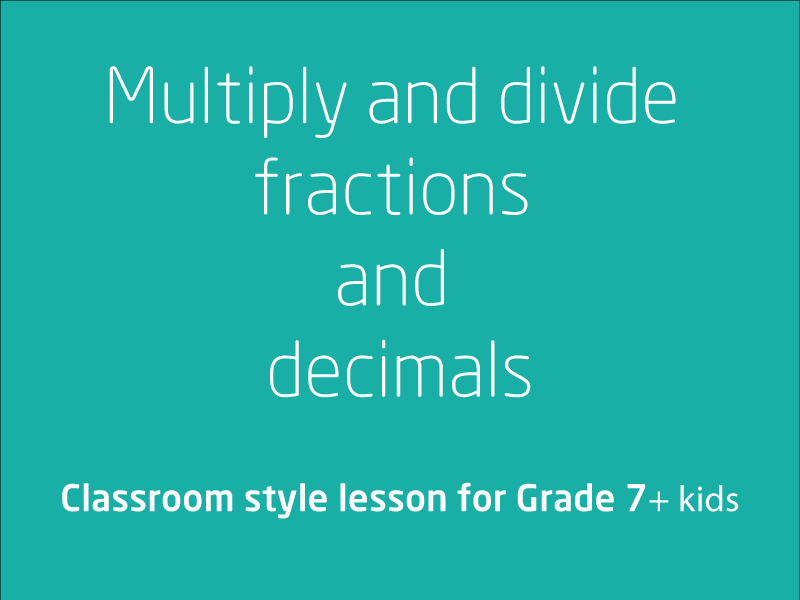### Multiply and divide fractions and decimals

In this video lesson, we will be looking at techniques that will help you take your multiplication and division skills involving fractions to a new level. This is a 3 part course. ...

3 videos
Intermediate difficulty
0 0 0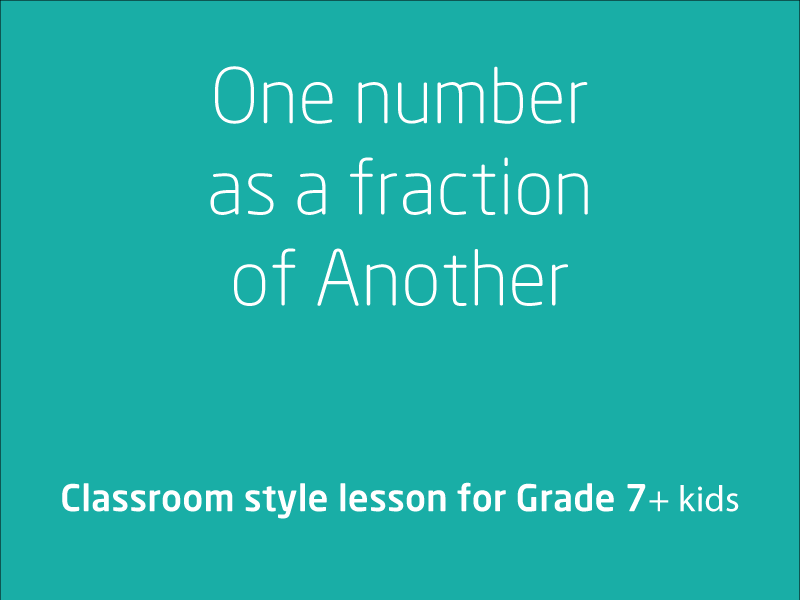### Expressing one number as a fraction of another

In this video lesson we will be working through Expressing one number as a fraction of another. We will be using authentic examples for the quantities to be expressed and...

1 videos
Intermediate difficulty
0 0 0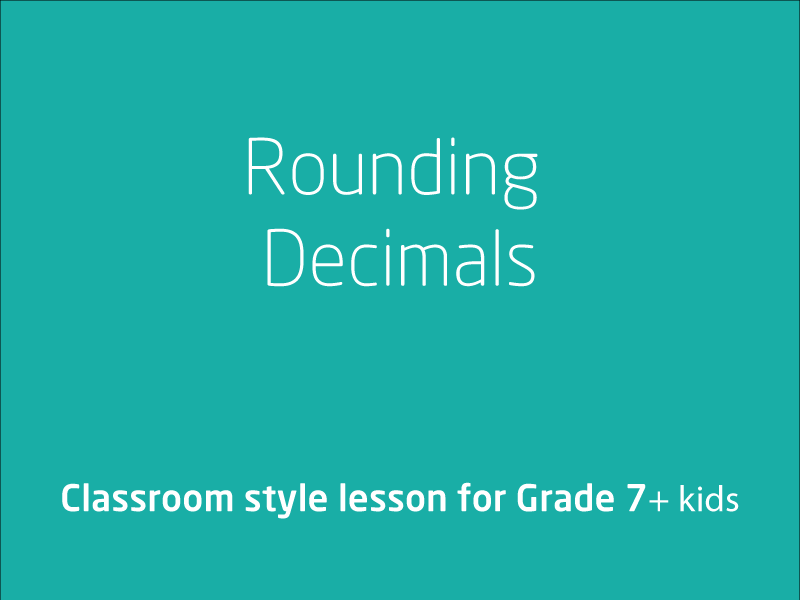### Rounding decimals to a specified number of decimal places

In this video lesson we will be exploring rounding decimals to a specific number of decimal places, using rounding to estimate the results of calculations with whole numbers a...

1 videos
Intermediate difficulty